Скачать презентацию EARTH SCIENCE Introduction Unit Day 1

10751817c6922aa8462aaa3f3bc36c65.ppt

• Количество слайдов: 30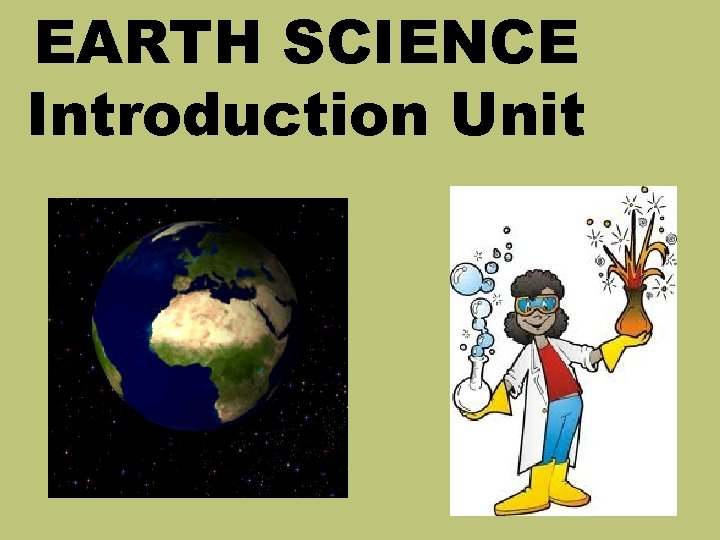EARTH SCIENCE Introduction Unit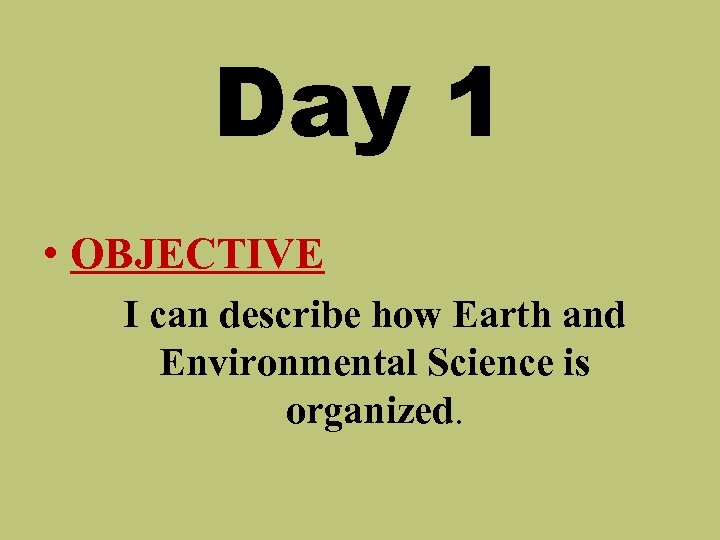Day 1 • OBJECTIVE I can describe how Earth and Environmental Science is organized.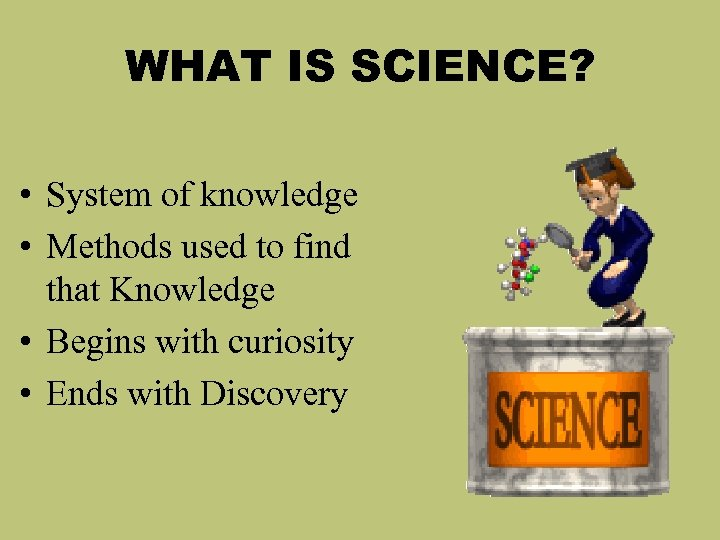WHAT IS SCIENCE? • System of knowledge • Methods used to find that Knowledge • Begins with curiosity • Ends with Discovery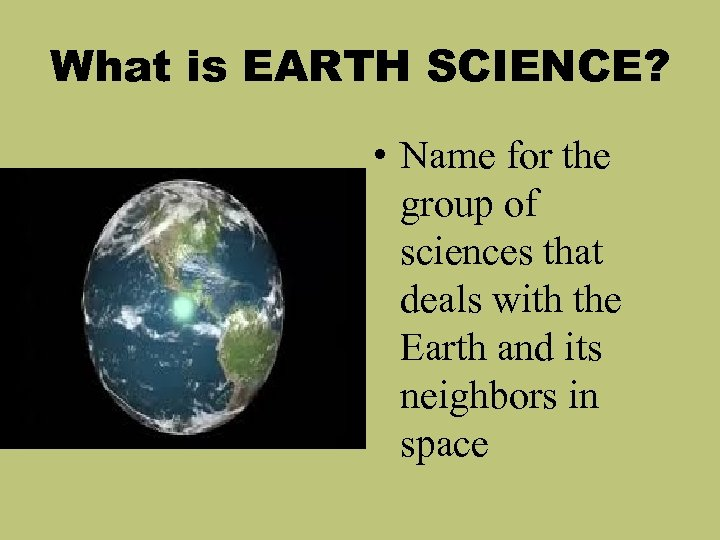What is EARTH SCIENCE? • Name for the group of sciences that deals with the Earth and its neighbors in space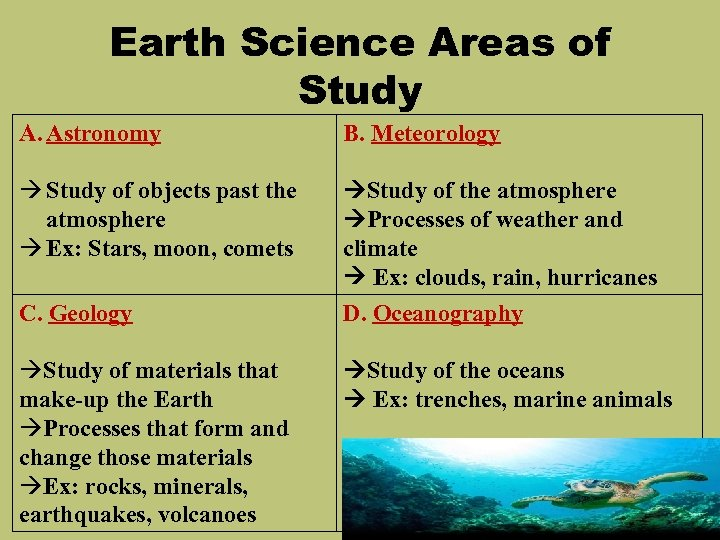Earth Science Areas of Study A. Astronomy B. Meteorology Study of objects past the atmosphere Ex: Stars, moon, comets Study of the atmosphere Processes of weather and climate Ex: clouds, rain, hurricanes C. Geology D. Oceanography Study of materials that make-up the Earth Processes that form and change those materials Ex: rocks, minerals, earthquakes, volcanoes Study of the oceans Ex: trenches, marine animals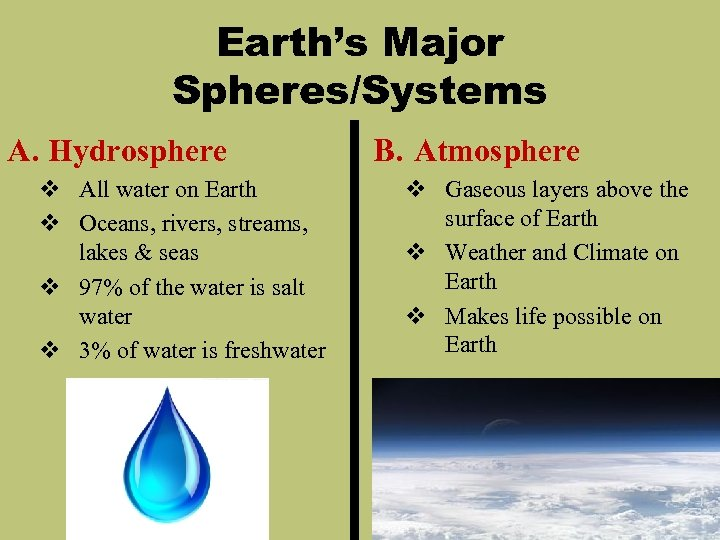Earth’s Major Spheres/Systems A. Hydrosphere v All water on Earth v Oceans, rivers, streams, lakes & seas v 97% of the water is salt water v 3% of water is freshwater B. Atmosphere v Gaseous layers above the surface of Earth v Weather and Climate on Earth v Makes life possible on Earth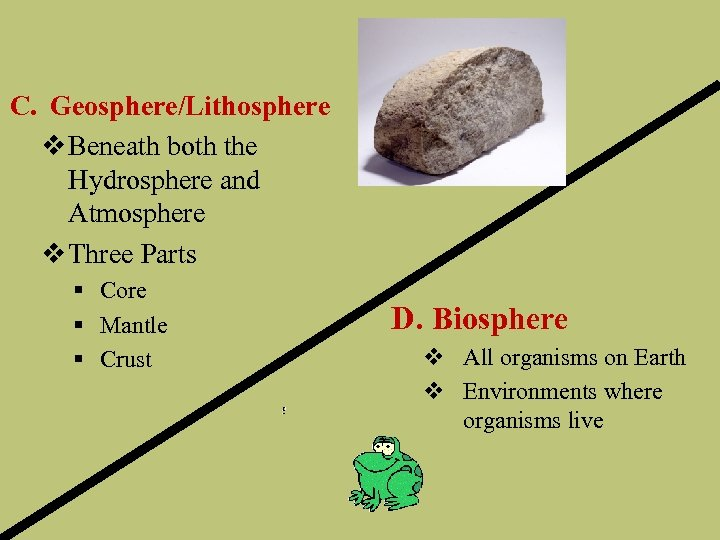C. Geosphere/Lithosphere v Beneath both the Hydrosphere and Atmosphere v Three Parts § Core § Mantle § Crust D. Biosphere v All organisms on Earth v Environments where organisms live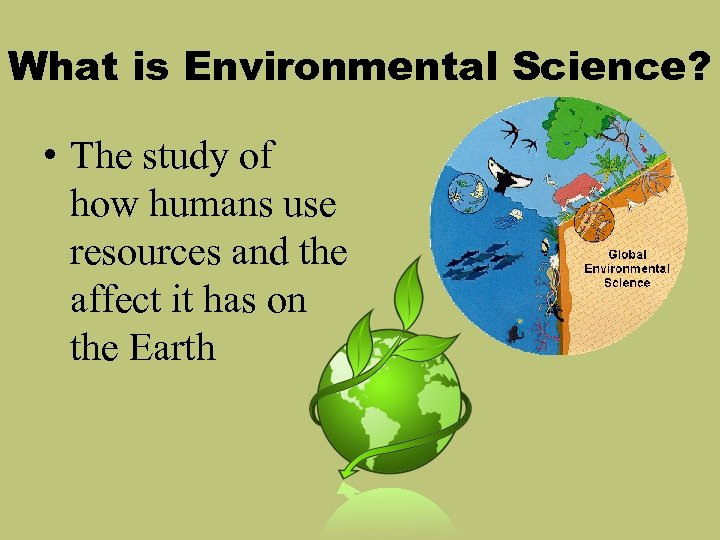What is Environmental Science? • The study of how humans use resources and the affect it has on the Earth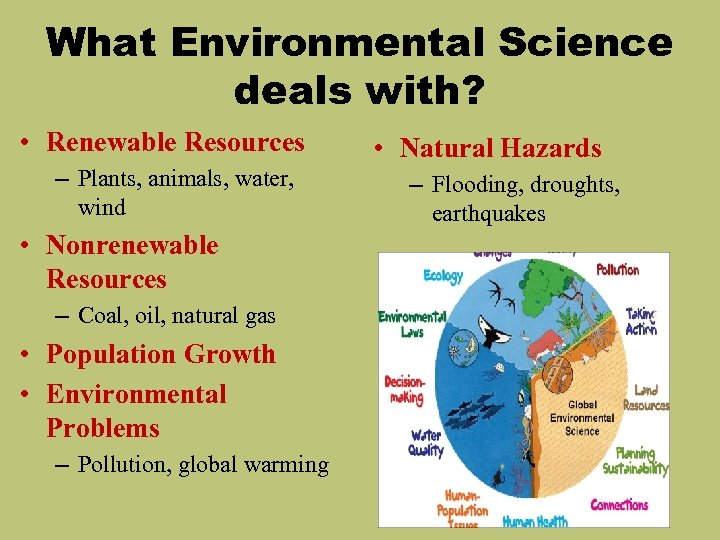What Environmental Science deals with? • Renewable Resources – Plants, animals, water, wind • Nonrenewable Resources – Coal, oil, natural gas • Population Growth • Environmental Problems – Pollution, global warming • Natural Hazards – Flooding, droughts, earthquakes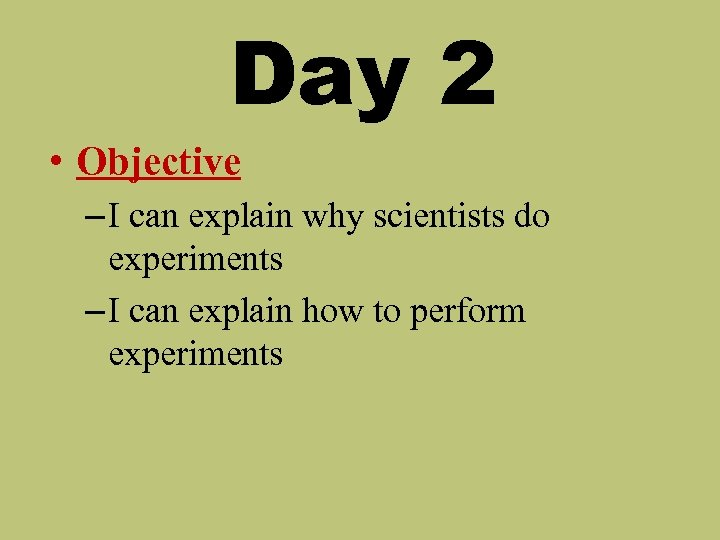Day 2 • Objective – I can explain why scientists do experiments – I can explain how to perform experiments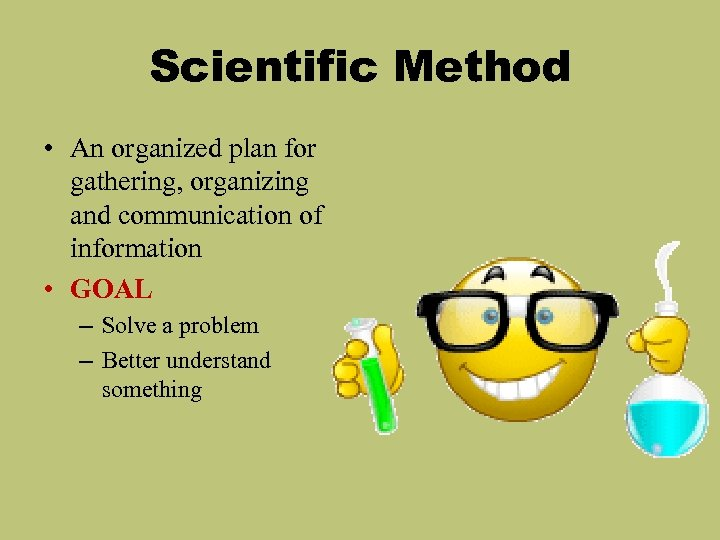Scientific Method • An organized plan for gathering, organizing and communication of information • GOAL – Solve a problem – Better understand something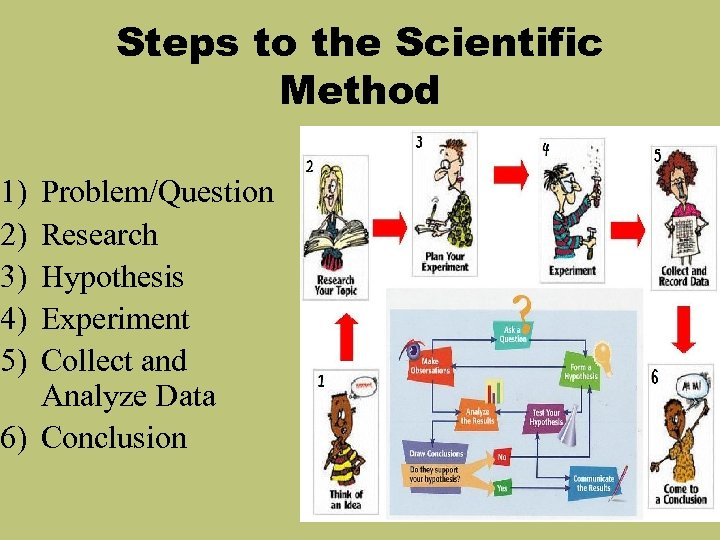Steps to the Scientific Method 1) 2) 3) 4) 5) Problem/Question Research Hypothesis Experiment Collect and Analyze Data 6) Conclusion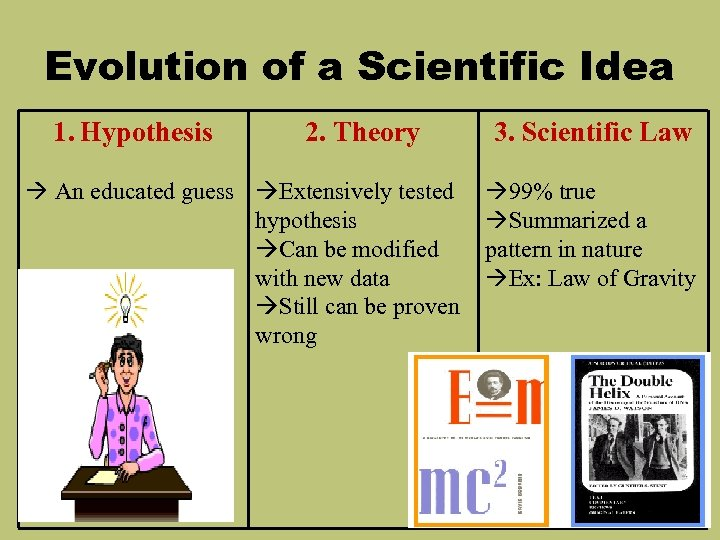Evolution of a Scientific Idea 1. Hypothesis 2. Theory An educated guess Extensively tested hypothesis Can be modified with new data Still can be proven wrong 3. Scientific Law 99% true Summarized a pattern in nature Ex: Law of Gravity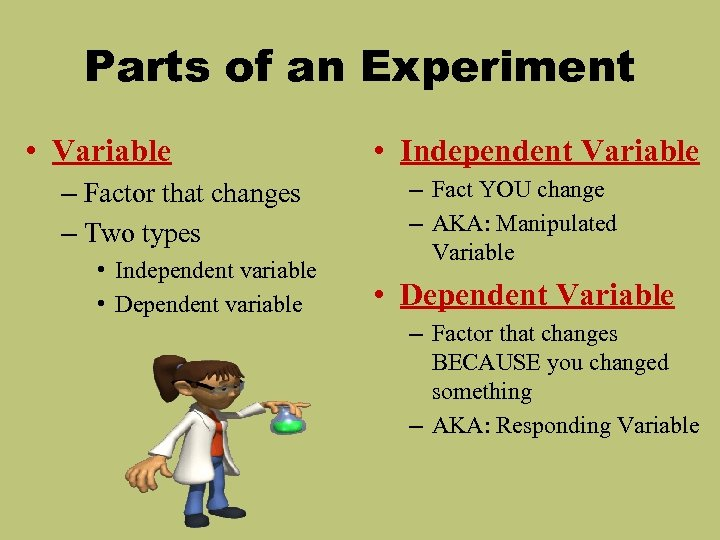Parts of an Experiment • Variable – Factor that changes – Two types • Independent variable • Dependent variable • Independent Variable – Fact YOU change – AKA: Manipulated Variable • Dependent Variable – Factor that changes BECAUSE you changed something – AKA: Responding Variable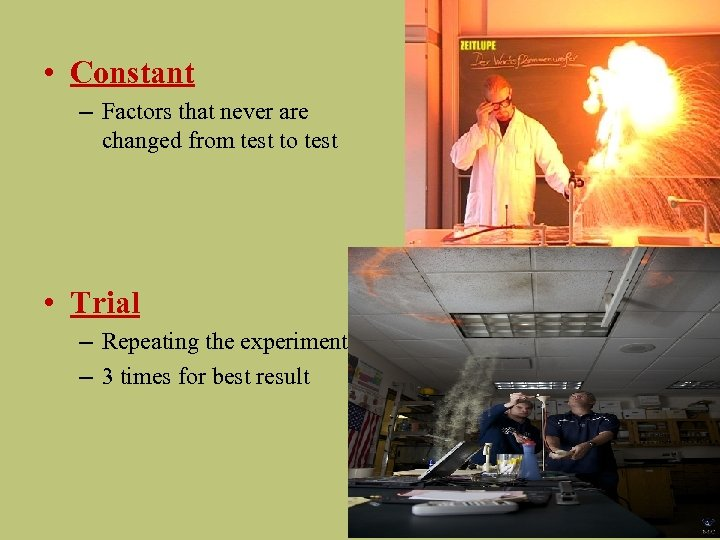• Constant – Factors that never are changed from test to test • Trial – Repeating the experiment – 3 times for best result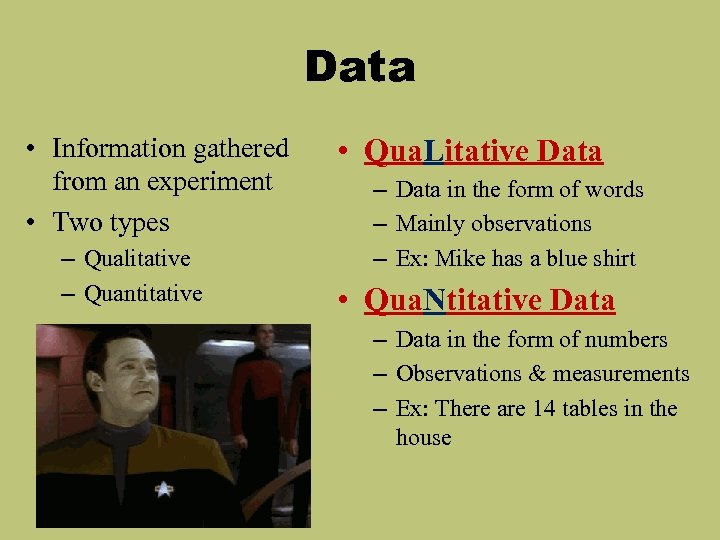Data • Information gathered from an experiment • Two types – Qualitative – Quantitative • Qua. Litative Data – Data in the form of words – Mainly observations – Ex: Mike has a blue shirt • Qua. Ntitative Data – Data in the form of numbers – Observations & measurements – Ex: There are 14 tables in the house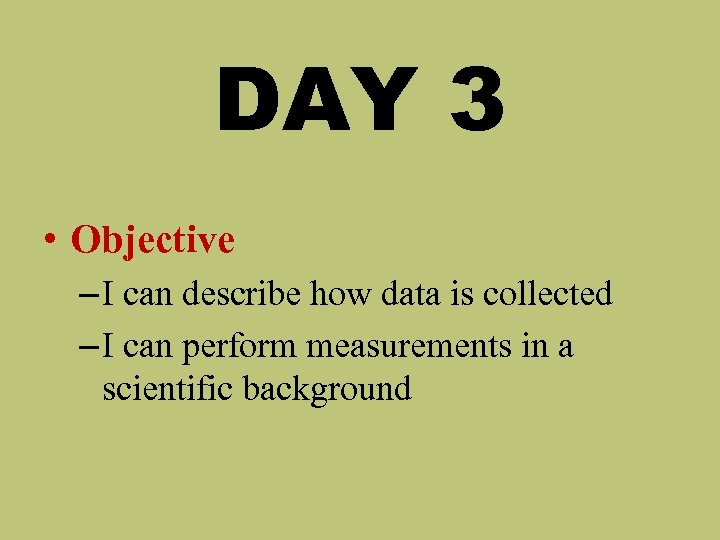DAY 3 • Objective – I can describe how data is collected – I can perform measurements in a scientific background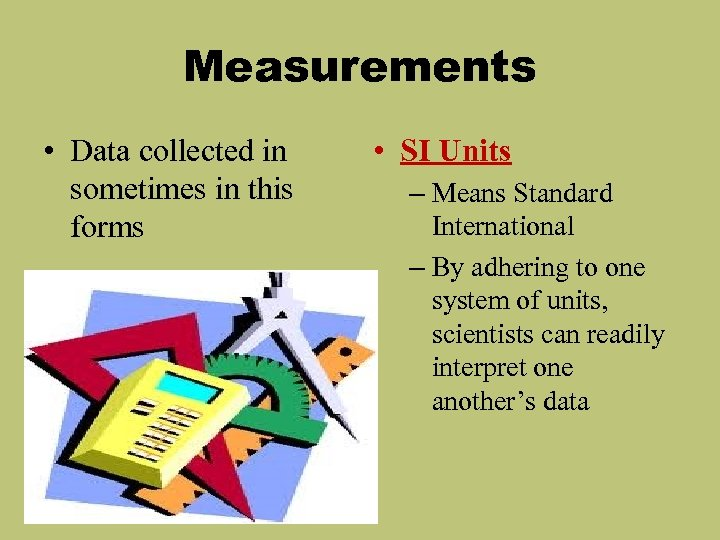Measurements • Data collected in sometimes in this forms • SI Units – Means Standard International – By adhering to one system of units, scientists can readily interpret one another’s data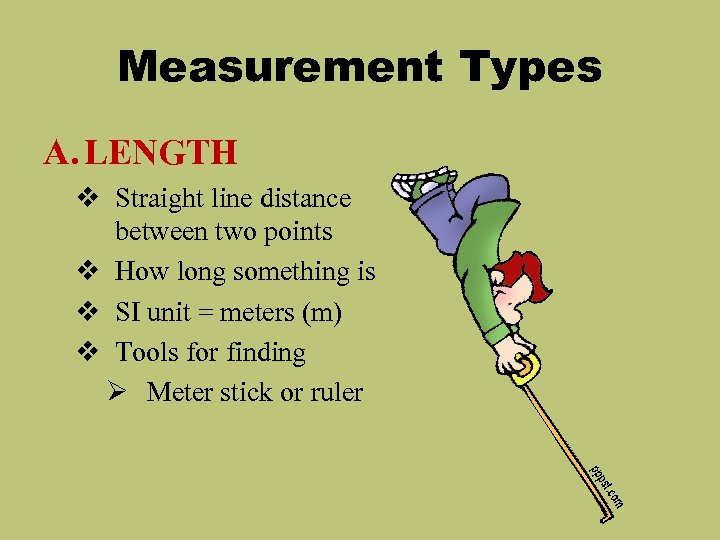Measurement Types A. LENGTH v Straight line distance between two points v How long something is v SI unit = meters (m) v Tools for finding Ø Meter stick or ruler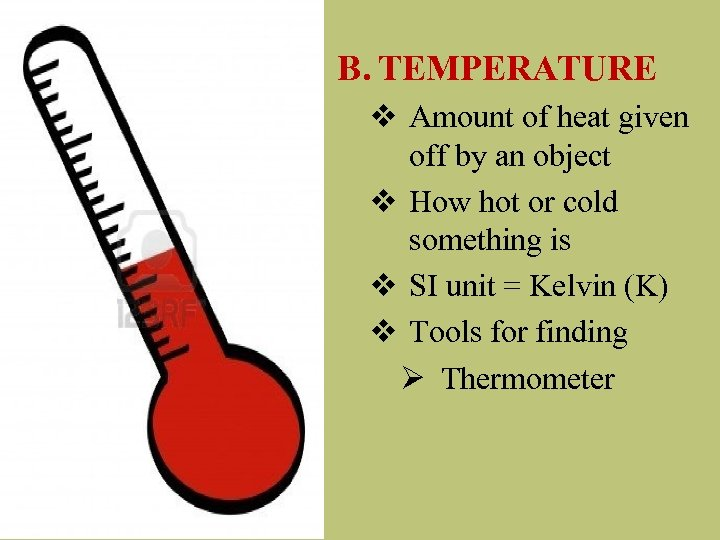B. TEMPERATURE v Amount of heat given off by an object v How hot or cold something is v SI unit = Kelvin (K) v Tools for finding Ø Thermometer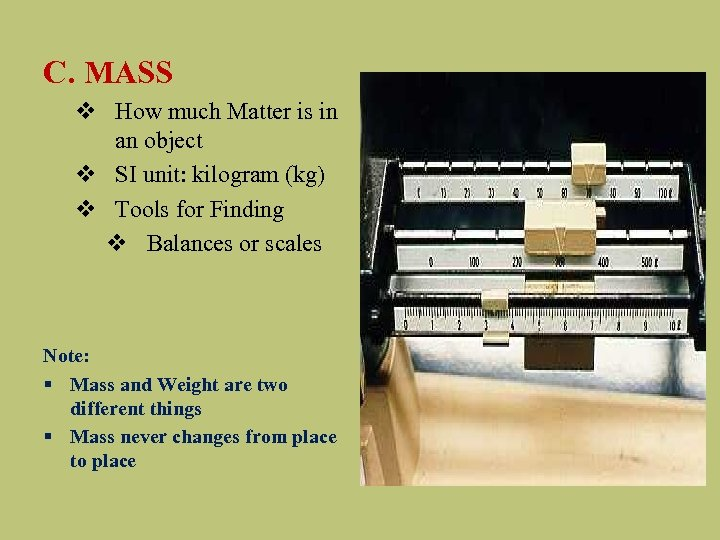C. MASS v How much Matter is in an object v SI unit: kilogram (kg) v Tools for Finding v Balances or scales Note: § Mass and Weight are two different things § Mass never changes from place to place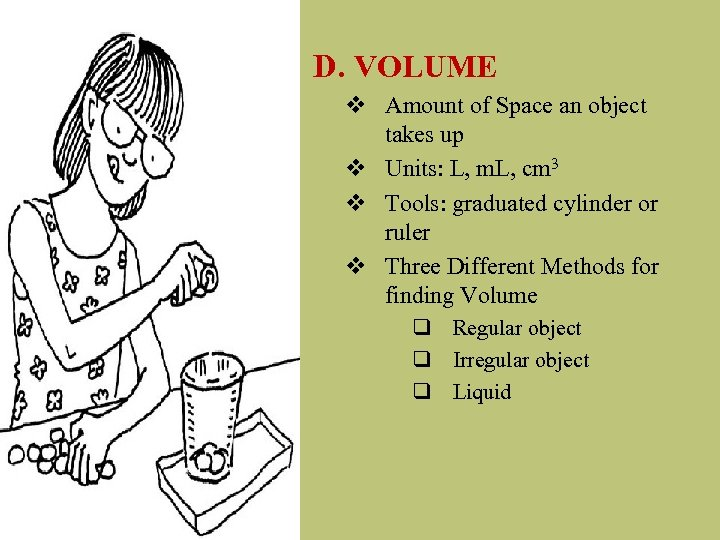D. VOLUME v Amount of Space an object takes up v Units: L, m. L, cm 3 v Tools: graduated cylinder or ruler v Three Different Methods for finding Volume q Regular object q Irregular object q Liquid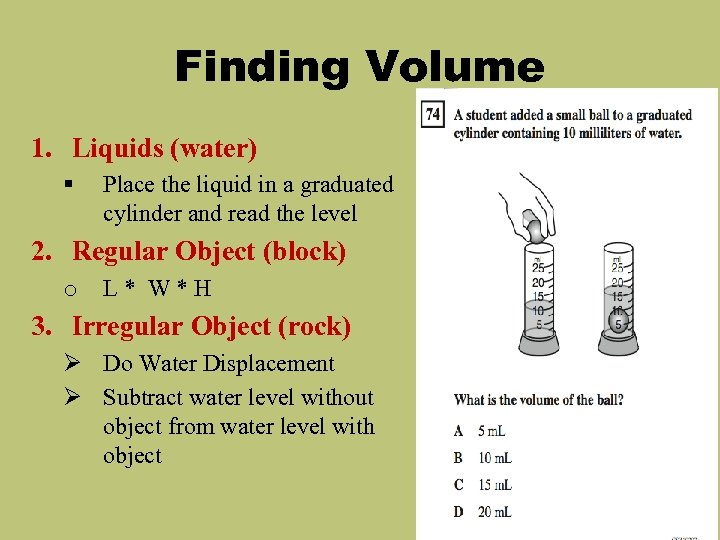Finding Volume 1. Liquids (water) § Place the liquid in a graduated cylinder and read the level 2. Regular Object (block) o L* W*H 3. Irregular Object (rock) Ø Do Water Displacement Ø Subtract water level without object from water level with object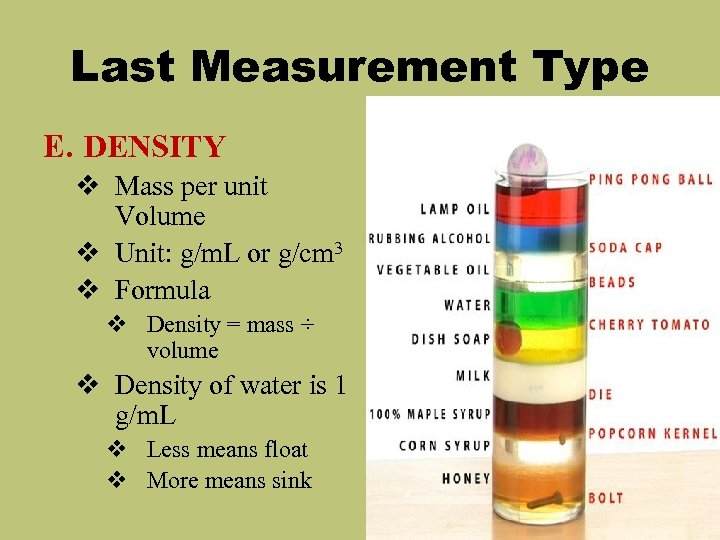Last Measurement Type E. DENSITY v Mass per unit Volume v Unit: g/m. L or g/cm 3 v Formula v Density = mass ÷ volume v Density of water is 1 g/m. L v Less means float v More means sink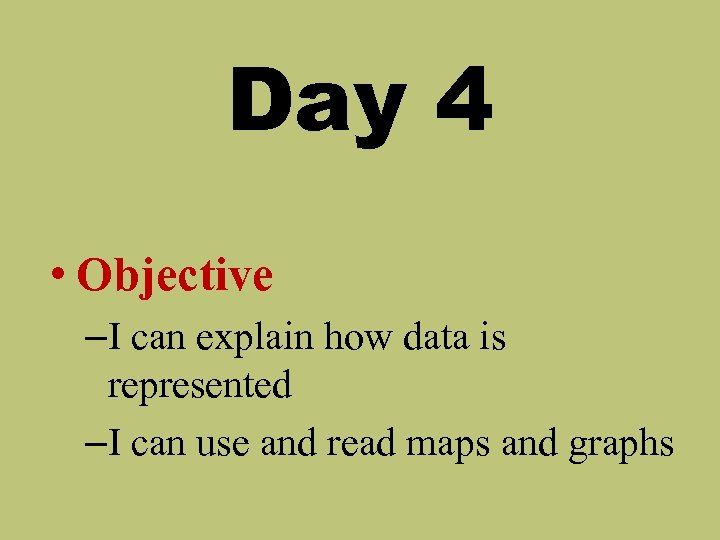Day 4 • Objective –I can explain how data is represented –I can use and read maps and graphs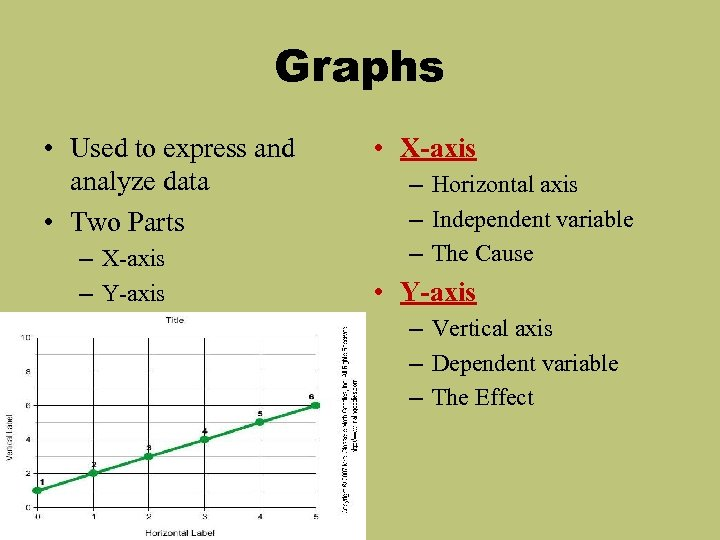Graphs • Used to express and analyze data • Two Parts – X-axis – Y-axis • X-axis – Horizontal axis – Independent variable – The Cause • Y-axis – Vertical axis – Dependent variable – The Effect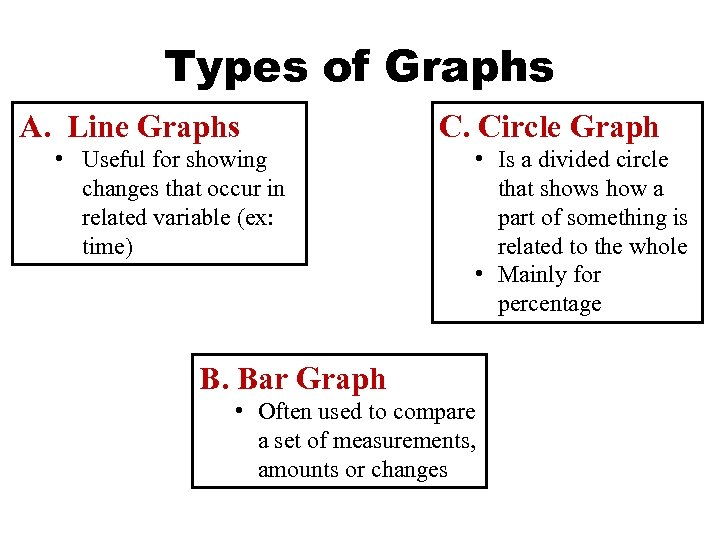Types of Graphs A. Line Graphs • Useful for showing changes that occur in related variable (ex: time) C. Circle Graph • Is a divided circle that shows how a part of something is related to the whole • Mainly for percentage B. Bar Graph • Often used to compare a set of measurements, amounts or changes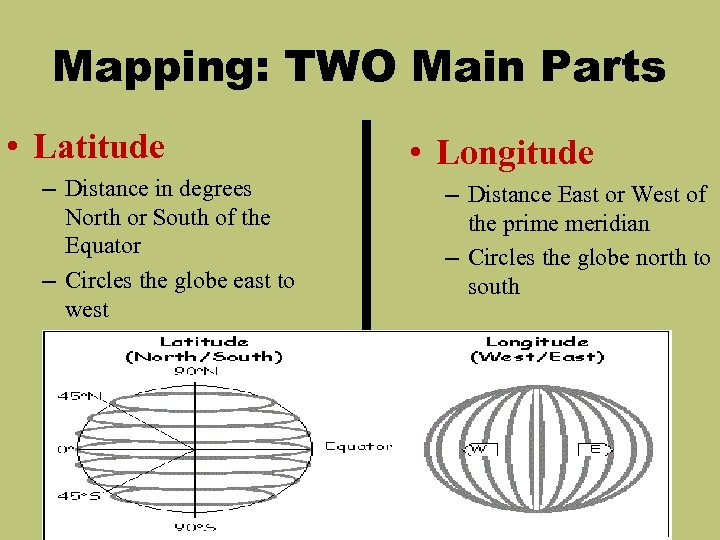Mapping: TWO Main Parts • Latitude – Distance in degrees North or South of the Equator – Circles the globe east to west • Longitude – Distance East or West of the prime meridian – Circles the globe north to south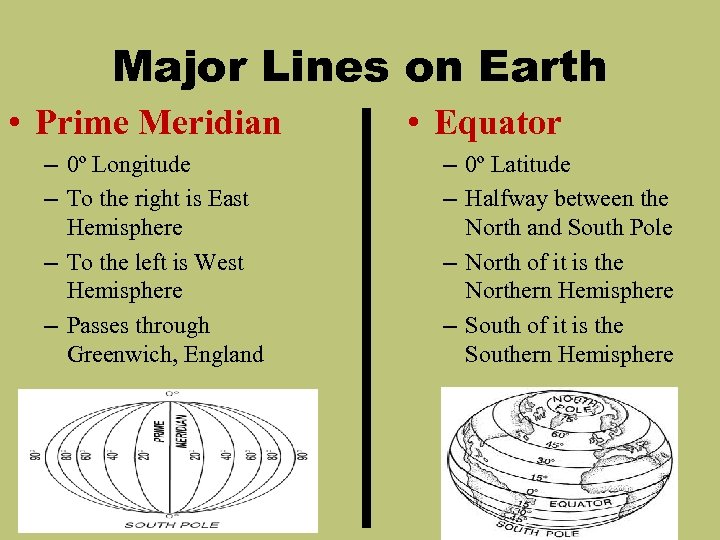Major Lines on Earth • Prime Meridian – 0º Longitude – To the right is East Hemisphere – To the left is West Hemisphere – Passes through Greenwich, England • Equator – 0º Latitude – Halfway between the North and South Pole – North of it is the Northern Hemisphere – South of it is the Southern Hemisphere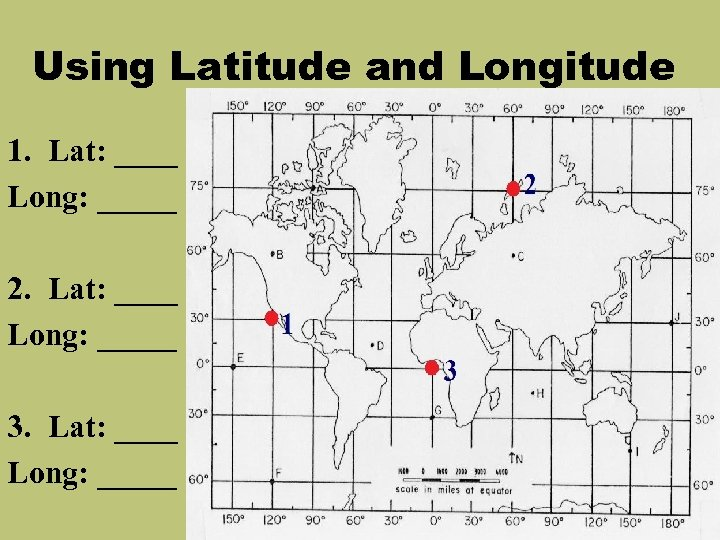Using Latitude and Longitude 1. Lat: ____ Long: _____ 2. Lat: ____ Long: _____ 3. Lat: ____ Long: _____